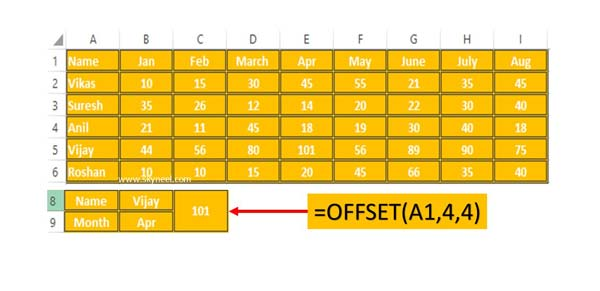# Easy Way to Use OFFSet Function in Excel

OFFSet Function in Excel allows you to return a reference to a range which is specified by number of rows and columns from a range of cell(s)

OFFSet is an important function of Excel OFFSet function in Excel allows you to quickly return a reference of a given range which is offset from the starting cell or range of cells which is specified by number of rows and columns. There are lot of inbuilt functions which is rarely used by different Excel users. But if you have better command on these functions then you can easily resolve different problems and make yourself as Excel expert.

## OFFSet Function in Excel

This function return a reference to a range which is specified by number of rows and columns from a range of cell(s). The reference which is returned may be a single cell or range of cells. You can specify number of rows or columns to be returned as per your need.

Syntax of OFFSet function in Excel

OFFSET(reference, rows, columns, [height], [width] )

reference: It is required and reference is the starting range from which the offset will be applied in active worksheet.

rows: Required. This is the number of rows, up to down that you want to apply as the offset to the range. It can be a positive or negative number.

columns: This is required entry of this function. The number of columns, to the left or right which you want to apply as the offset to the range. It can be a positive or negative number.

height: It’s an optional entry. It is the height, in number of rows that you want the returned range to be. Height must be positive number.

width: This is another optional entry. The width is the number of columns that you want the returned range to be. Width must be positive number

### Steps to Use OFFSet Function in Excel

If you want to use OFFSet function in Excel then you have to take following given simple steps, have a look.Step 1: To understand OFFSet function in Excel let’s prepare the following worksheet.

Step 2: In this worksheet you want to know Vijay total points for the month of Apr with the help of OFFSet function. To do this job you must have to use given function =OFFSET(A1,4,4) and press enter to get the result.

OFFSet is the name of function.

A1: It is reference number of starting location from which the offset will be applied in active worksheet.

4: This is the row number that you want to apply as the offset to the range. It may be a positive or negative number.

4: It is the columns number that to apply as the offset to the range. It is also a positive or negative number.

After reading this simple example you can easily use OFFSet Function in Excel. If you have any doubt then please put your doubt in the comment box.